Question

# A multiple choice test has 10 questions each of which has 4 possible answers, only one...

A multiple choice test has 10 questions each of which has 4 possible answers, only one of which is correct. If Judy, who forgot to study for the test, guesses on all questions, what is the probability that she will answer exactly 3 questions correctly?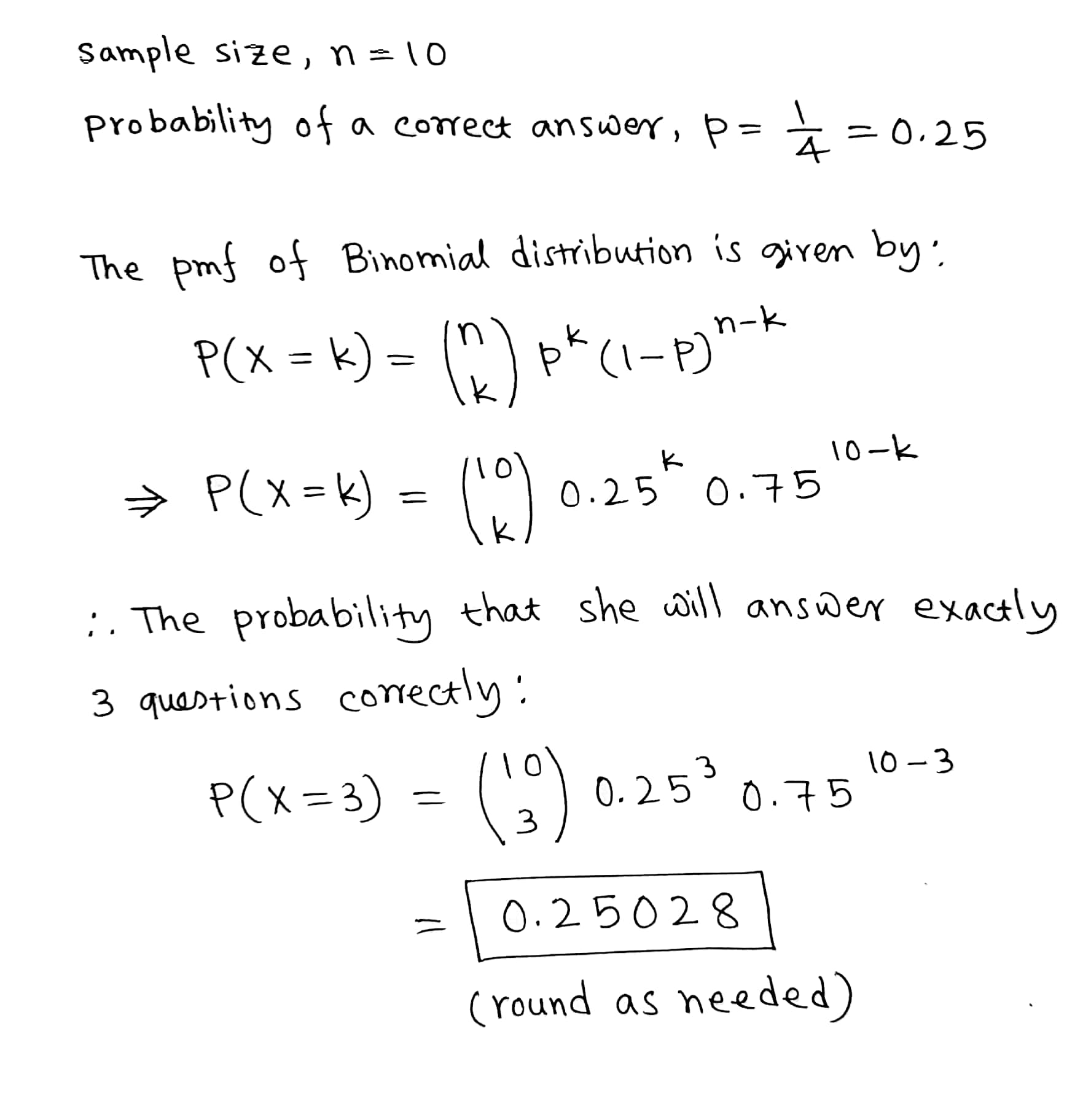#### Earn Coins

Coins can be redeemed for fabulous gifts.

Similar Homework Help Questions
• ### Find the indicated probability, A multiple choice test has 8 questions each of which has 4...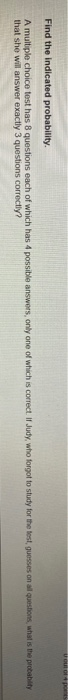Find the indicated probability, A multiple choice test has 8 questions each of which has 4 possible answers, only one of which is correct. Il Judy, who forgot to study for the test guesses on all questions, what is the probability that she will answer exactly 3 questions correctly?

• ### find the indicated probability

A multiple choice test has 10 questions each of which has 4 possible answers, only one of which is correct. If Judy, who forgot to study for the test, guesses on allquestions, what is the probability that she will answer exactly 3 questions correctly?a-0.2816b-0.0021c-0.5006d-0.0156e-0.2503

• ### 10. A multiple-choice test has 30 questions and each one has five possible answers, of which one...

10. A multiple-choice test has 30 questions and each one has five possible answers, of which one is correct. If all answers were guesses, find the probability ofgetting exactly four correct answers. (Points : 3).0604.1325.2552.8000

• ### 10 multiple choice questions each with three possible answers only one of which is correct

10 multiple choice questions each with three possible answers only one of which is correct. A student guesses the answer to every question. (a)What is the probability that the student will answer the first six questions correctly and the rest incorrectly?(b)What is the probability that the student will answer the 1st,2nd,4th,6th,7th,and 10th questions correctly and the rest incorrectly?(c)How many ways can the student get exactly 6 correct answers?(d)What is the probability that the student answers exactly 6 questions correctly?Thank you...

• ### A multiple choice test consists of 60 questions. each question has 4 possible answers of which...

A multiple choice test consists of 60 questions. each question has 4 possible answers of which one is correct. If all answers are random guesses, estimate the probability that of 140 randomly selected seeds, exactly 100 will germinate

• ### 4 0.0256 Find the indicated probability. 5) A multiple choice test has 8 questions each of...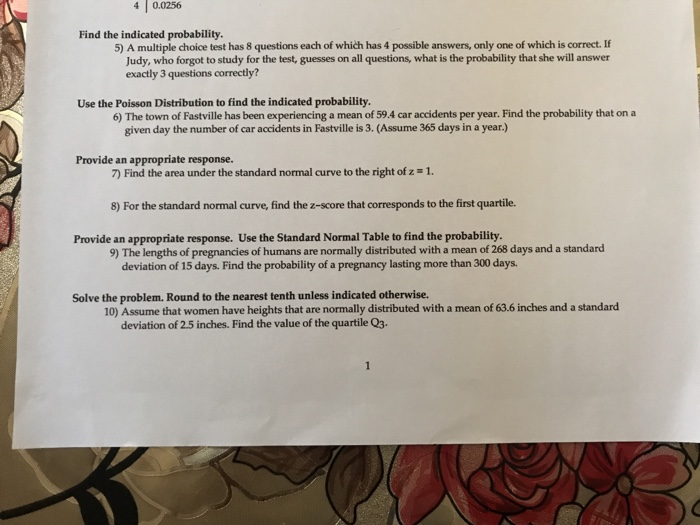4 0.0256 Find the indicated probability. 5) A multiple choice test has 8 questions each of which has 4 possible answers, only one of which is correct. If Judy, who forgot to study for the test, guesses on all questions, what is the probability that she will answer exactly 3 questions correctly? Use the Poisson Distribution to find the indicated probability. 6) The town of Fastville has been experiencing a mean of 59.4 car accidents per year. Find the probability...

• ### 5) A student takes a multiple choice test with 12 questions, Each question has 4 possible...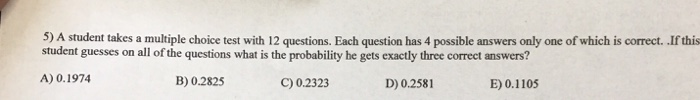5) A student takes a multiple choice test with 12 questions, Each question has 4 possible answers only one of which is correct. .If this student guesses on all of the questions what is the probability he gets exactly three correct answers? A) 0.1974 C) 0.2323 B) 0.2825 D) 0.2581 E) 0.1105

• ### (5 points) A multiple-choice examination has 15 questions, each with 5 possible answers only one ...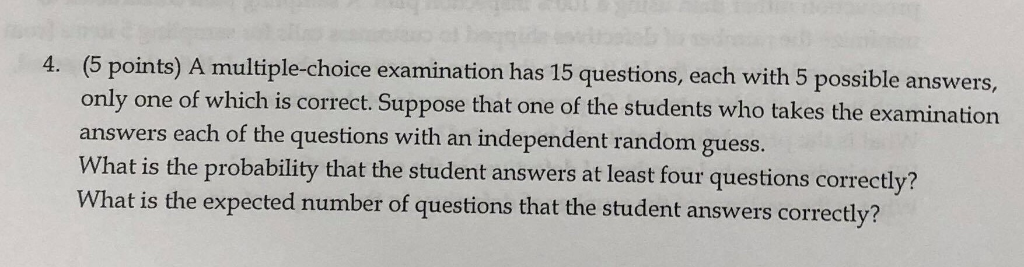(5 points) A multiple-choice examination has 15 questions, each with 5 possible answers only one of which is correct. Suppose that one of the students who takes the examination answers each of the questions with an independent random guess. What is the probability that the student answers at least four questions correctly? What is the expected number of questions that the student answers correctly? 4. (5 points) A multiple-choice examination has 15 questions, each with 5 possible answers only one...

• ### A multiple-choice test has 20 questions. Each question has 4 possible answers, of which only one ...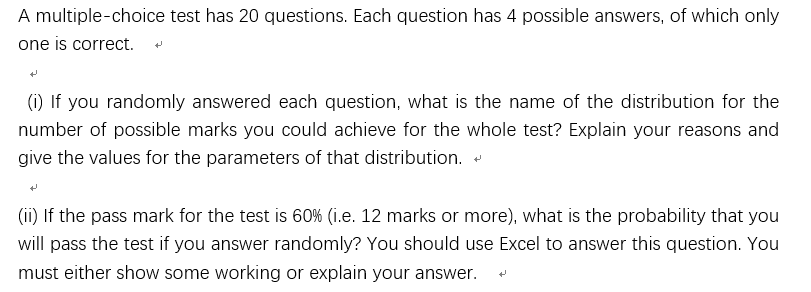A multiple-choice test has 20 questions. Each question has 4 possible answers, of which only one is correct. (i) If you randomly answered each question, what is the name of the distribution for the number of possible marks you could achieve for the whole test? Explain your reasons and give the values for the parameters of that distribution.* (ii) If the pass mark for the test is 60% (i.e. 12 marks or more), what is the probability that you will...

• ### Sa Find the indicated probability 4 points A multiple choice test has 3 questions each of...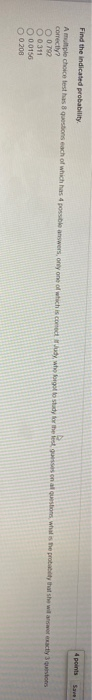Sa Find the indicated probability 4 points A multiple choice test has 3 questions each of which has 4 possible anwers only one of which is comedy who forgot to stay for the last on alustion what is the probably that she will ansactly to correctly O 0790 O 0311 0.0156 O 0 200